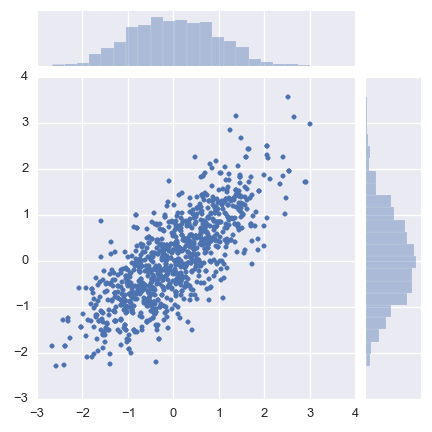# Sampyl: MCMC samplers in Python¶

Release v0.3

Sampyl is a Python library implementing Markov Chain Monte Carlo (MCMC) samplers in Python. It’s designed for use in Bayesian parameter estimation and provides a collection of distribution log-likelihoods for use in constructing models.

Our goal with Sampyl is allow users to define models completely with Python and common packages like Numpy. Other MCMC packages require learning new syntax and semantics while all that is really needed is a function that calculates $$\log{P(X)}$$ for the sampling distribution.

Sampyl allows the user to define a model any way they want, all that is required is a function that calculates log P(X). This function can be written completely in Python, written in C/C++ and wrapped with Python, or anything else a user can think of. For samplers that require the gradient of P(X), such as NUTS, Sampyl can calculate the gradients automatically with autograd.

To show you how simple this can be, let’s sample from a 2D correlated normal distribution.

# To use automatic gradient calculations, use numpy (np) provided
# by autograd through Sampyl
import sampyl as smp
from sampyl import np
import seaborn

icov = np.linalg.inv(np.array([[1., .8], [.8, 1.]]))
def logp(x, y):
d = np.array([x, y])
return -.5 * np.dot(np.dot(d, icov), d)

start = {'x': 1., 'y': 1.}
nuts = smp.NUTS(logp, start)
chain = nuts.sample(1000)

seaborn.jointplot(chain.x, chain.y, stat_func=None)